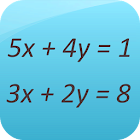Linear Equation System Solver

• 검색 유형

모든 Android 애플리케이션 카테고리

모든 Android 게임 카테고리# Linear Equation System Solver

by: 3.4K 7.4

7.4 사용자
평가 등급

## 스크린샷

설명

This app is a free math calculator which is able to solve systems of linear equations.

You can solve:
-Systems of two variables and two equations
-Systems of three variables and two equations
-Systems of three variables and three equations
-Systems of four variables and two equations
-Systems of four variables and three equations
-Systems of four variables and four equations
-Systems of four variables and four equations
-Systems with n variables and m equations (more than 4)

Best mathematical tool for school and college! If you are a student, it will help you to learn linear algebra!
Note: Linear equation systems play an important role in engineering, physics, chemistry, computer science, and economics.

: 3.371 리뷰

"우수"

7.4